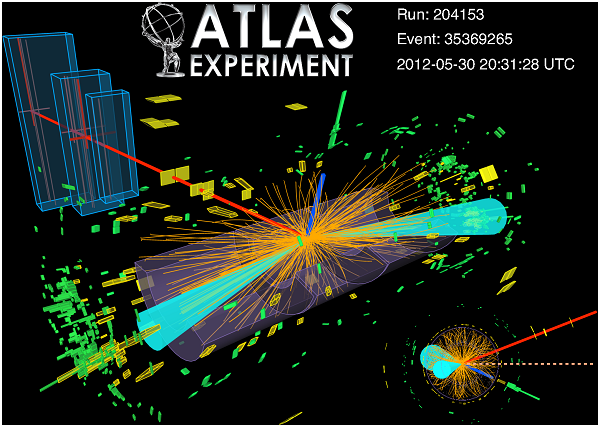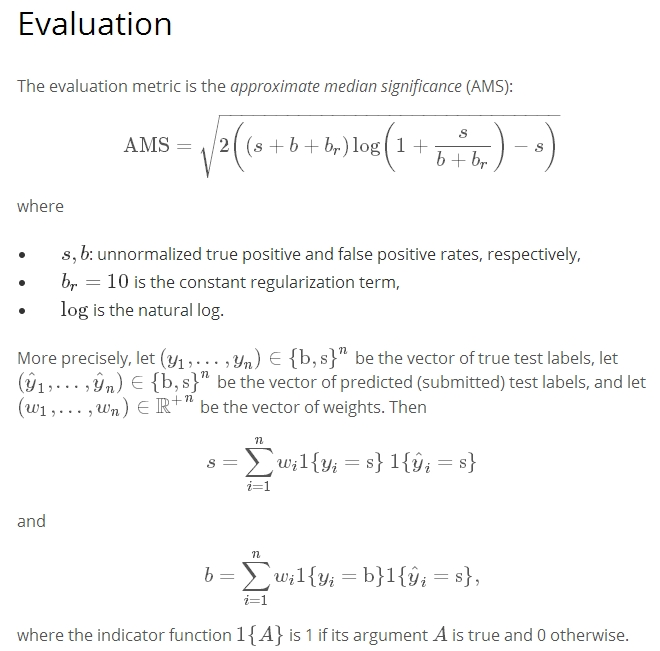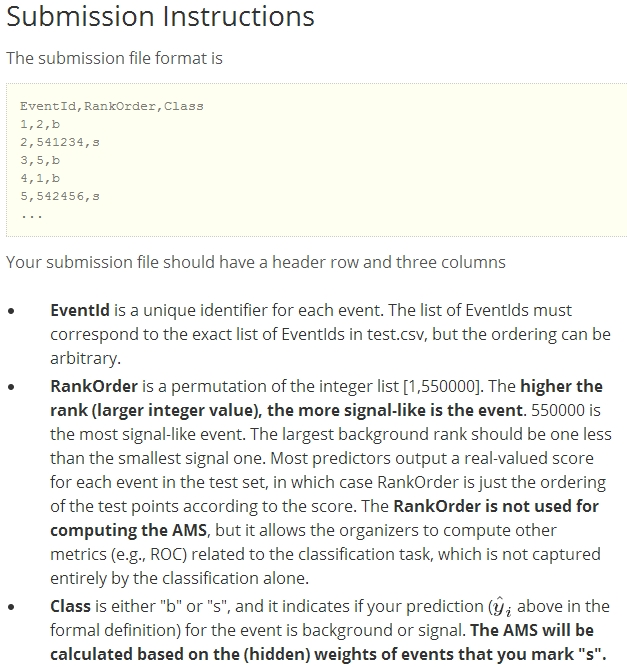Higgs Boson Machine Learning Challenge with Pandas and Scikit Learn¶

In :
from IPython.core.display import Image
Image('https://kaggle2.blob.core.windows.net/competitions/kaggle/3887/media/ATLASEXP_image.png')
Out:Kaggle is a place where peope meet to work on Data Mining problems derived from science Visit: https://www.kaggle.com/c/higgs-boson for more information

This notebook is based on Darin Baumgartel, you can find his original implementation here: https://dbaumgartel.wordpress.com/author/dbaumgartel/

For This Classification Problem he uses Gradient Boosted Trees. This Machine Learning Technique is described in a detailed way in another ipython notebook here: http://nbviewer.ipython.org/urls/s3.amazonaws.com/datarobotblog/notebooks/gbm-tutorial.ipynb

This notebook represents my personal notes on the implementation by Darin Baumgartel. He had the idea, i followed him, but changed the way of representation of the data by using Pandas

In :
import numpy as np
import scipy as sc
import pandas as pd
import sklearn as sk
import matplotlib.pyplot as plt
from sklearn.ensemble import GradientBoostingClassifier as GBC
import os
import pickle
os.chdir("C:\python_usb_sync\kaggle\Higgs_Boson")

In :
print('Python version ' + sys.version)
print('Pandas version ' + pd.__version__)
print('Numpy version ' + np.__version__)
print('Scikit version ' + sk.__version__)
Python version 3.3.2 (v3.3.2:d047928ae3f6, May 16 2013, 00:06:53) [MSC v.1600 64 bit (AMD64)]
Pandas version 0.12.0
Numpy version 1.7.1
Scikit version 0.14.1
In [ ]:

In :
import warnings
warnings.filterwarnings("ignore", category=DeprecationWarning)
In :
pd.set_option('display.notebook_repr_html', True)
In :
In :
train_data.iloc[:10,:5]
Out:
EventId DER_mass_MMC DER_mass_transverse_met_lep DER_mass_vis DER_pt_h
0 100000 138.470 51.655 97.827 27.980
1 100001 160.937 68.768 103.235 48.146
2 100002 -999.000 162.172 125.953 35.635
3 100003 143.905 81.417 80.943 0.414
4 100004 175.864 16.915 134.805 16.405
5 100005 89.744 13.550 59.149 116.344
6 100006 148.754 28.862 107.782 106.130
7 100007 154.916 10.418 94.714 29.169
8 100008 105.594 50.559 100.989 4.288
9 100009 128.053 88.941 69.272 193.392
In :
In :
In :
train_data.iloc[:10,31:34]
Out:
Weight Label class_int
0 0.002653 s 1
1 2.233584 b 0
2 2.347389 b 0
3 5.446378 b 0
4 6.245333 b 0
5 0.083414 b 0
6 0.002653 s 1
7 0.018636 s 1
8 5.296003 b 0
9 0.001502 s 1
In :
#from sklearn import svm

We will use 90% of the training data to train our model 10% will be used to validate it. Before we make a submission we should test our model, this is why we keep 10% for validation

In :
train_ind=int(0.9*train_data.shape)
In :
train_ind
Out:
225000
In :
train_data.shape
Out:
(250000, 34)

rat is the Ration

In :
rat=float(train_ind)/int(train_data.shape)
rat
Out:
0.9
In :
var_no=31#number var to input
var_start=0

#these where used just for some testing, not used in this version
no_train=10000#trainings dataset to input
predict_to=15000#show prediction up to

#setting up our data for testing, validating and the weights that are used to calculate a metric
#later
x_tr=train_data.iloc[:train_ind,1:var_no].values#event ID not in training please correct!
y_tr=train_data.iloc[:train_ind,33].values
x_val=train_data.iloc[train_ind:,1:var_no].values
y_val=train_data.iloc[train_ind:,33].values

#weights
w_tr=train_data.iloc[:train_ind,var_no].values
w_val=train_data.iloc[train_ind:,var_no].values

The Next Cell runs about 4 minutes on my laptop

If you want to keep the model as it is, you can use pickle which lets you save in a binary way.

If you already trained your model you can load it up now with the following command: remember to comment your scikit command in this case

In [ ]:

#my_object_file = open('classifiers.pkl', 'rb')
#my_object_file.close()
In :
#clf = svm.SVC(probability=True)
#clf.fit(train_data.iloc[:no_train,:var_no].values,train_data.iloc[:no_train,33].values,sample_weight=train_data.iloc[:no_train,31].values)#sample_weight=train_data.iloc[:no_train,31].values

clf = GBC(n_estimators=50, max_depth=5,min_samples_leaf=200,max_features=10,verbose=1)
clf.fit(x_tr,y_tr)
Iter       Train Loss   Remaining Time
1           1.2260            5.01m
2           1.1677            4.76m
3           1.1239            4.57m
4           1.0804            4.47m
5           1.0430            4.45m
6           1.0109            4.38m
7           0.9815            4.27m
8           0.9567            4.19m
9           0.9383            4.10m
10           0.9202            3.98m
20           0.8165            3.00m
30           0.7758            2.06m
40           0.7530            1.04m
50           0.7407            0.00s
Out:
max_depth=5, max_features=10, min_samples_leaf=200,
min_samples_split=2, n_estimators=50, random_state=None,
subsample=1.0, verbose=1)

The following code shows how to save the classifier for later use¶

In :
###put it to file to load it later
#pickle_out = open('classifiers.pkl', 'wb')
#pickle.dump(clf, pickle_out)
#pickle_out.close()
In :
y_tr_pre=clf.predict(x_tr)#makes a prediction for our training data

A '1' is a prediction for a "signal like event" a '0' represents a "background signal"¶

You can clearly see that the model makes some wrong decisions in the beginnin of data, but the amount of data is huge so do not be concerned

In :
y_tr_pre[:15]
Out:
array([0, 1, 0, 0, 0, 0, 1, 1, 0, 1, 0, 1, 1, 0, 0], dtype=object)
In :
y_tr[:15]
Out:
array([1, 0, 0, 0, 0, 0, 1, 1, 0, 1, 0, 0, 0, 0, 0], dtype=object)

If it estimates a probability above 0.5 it marks the dataset as a "signal like event"¶

In :
prob_pre_tr=clf.predict_proba(x_tr)[:,1]#just takes the second column the first is the 'reverse probab.'
prob_pre_val=clf.predict_proba(x_val)[:,1]

Look at the following two cells and you understand the concepts

In :
prob_pre_tr[:15]
Out:
array([ 0.43254476,  0.52566156,  0.13116018,  0.08748292,  0.06219945,
0.12732228,  0.74897787,  0.85925578,  0.44136698,  0.71589157,
0.05965795,  0.55914471,  0.68378702,  0.20416104,  0.0370057 ])
In :
y_tr_pre[:15]
Out:
array([0, 1, 0, 0, 0, 0, 1, 1, 0, 1, 0, 1, 1, 0, 0], dtype=object)
In :
pcut = np.percentile(prob_pre_tr,88)# i take 88 percent as the new cut for marking a "SLE"
In :
pcut#everything above this value is considered a "Signal"
Out:
0.82141799300687846
In :
Yhat_tr = prob_pre_tr > pcut
Yhat_val = prob_pre_val > pcut
In :
Yhat_tr[0:15]
Out:
array([False, False, False, False, False, False, False,  True, False,
False, False, False, False, False, False], dtype=bool)
In :
y_tr
Out:
array([1, 0, 0, ..., 1, 0, 0], dtype=object)
In :
w_tr[:15]
Out:
array([  2.65331134e-03,   2.23358449e+00,   2.34738894e+00,
5.44637821e+00,   6.24533269e+00,   8.34140313e-02,
2.65331134e-03,   1.86361167e-02,   5.29600299e+00,
1.50187016e-03,   2.29950374e+00,   3.07169524e-01,
1.68161144e+00,   2.18389154e+00,   2.15119867e+00])

The Metric 'penalizes' according to the weight that is linked with an event.¶

In :
Image('http://i.imgur.com/Hflz2lG.jpg')
Out:In :
TruePositive_train = w_tr*(y_tr==1.0)*(1.0/rat)
TrueNegative_train = w_tr*(y_tr==0.0)*(1.0/rat)
TruePositive_valid = w_val*(y_val==1.0)*(1.0/(1-rat))
TrueNegative_valid = w_val*(y_val==0.0)*(1.0/(1-rat))
In :
s_train = sum ( TruePositive_train*(Yhat_tr==1.0) )#here only the "cases" are summed where prediction and "real" signal come together
b_train = sum ( TrueNegative_train*(Yhat_tr==1.0) )#...
s_valid = sum ( TruePositive_valid*(Yhat_val==1.0) )
b_valid = sum ( TrueNegative_valid*(Yhat_val==1.0) )
In :
print('Calculating AMS score for a probability cutoff pcut=',pcut)
def AMSScore(s,b): return  math.sqrt (2.*( (s + b + 10.)*math.log(1.+s/(b+10.))-s))
print( '   - AMS based on %s %% training   sample:' % (rat*100),AMSScore(s_train,b_train))
print('   - AMS based on %s %% validation sample:' % ((1-rat)*100),(AMSScore(s_valid,b_valid)))
Calculating AMS score for a probability cutoff pcut= 0.821417993007
- AMS based on 90.0 % training   sample: 3.3691789739078146
- AMS based on 9.999999999999998 % validation sample: 3.1431799979664627

Reading in the test data so we can make a prediction with our model¶

In :
X_val=val_data.iloc[:,1:var_no].values
Y_val=clf.predict_proba(X_val)[:,1]
In :
val_data.iloc[:5,:5]
Out:
EventId DER_mass_MMC DER_mass_transverse_met_lep DER_mass_vis DER_pt_h
0 350000 -999.000 79.589 23.916 3.036
1 350001 106.398 67.490 87.949 49.994
2 350002 117.794 56.226 96.358 4.137
3 350003 135.861 30.604 97.288 9.104
4 350004 74.159 82.772 58.731 89.646

We prepare a new Dataframe now. Columns we need are: probab_s which is the Probability fo a Signal like Event and the following...

In :

Yhat_val_wild = Y_val > pcut

#DataFrames
In :
fin.iloc[:5,:]
Out:
EventId probab_s decision
0 350000 0.028652 0
1 350001 0.159228 0
2 350002 0.484027 0
3 350003 0.845426 1
4 350004 0.044841 0

Now we sort the data because we need to give the Data sets a wheight according to this probabilities, we will look at the instructions...

In :
sort_pro=fin.sort(['probab_s'],ascending=True)
In :
Out:
EventId probab_s decision
96821 446821 0.016244 0
103392 453392 0.016377 0
71192 421192 0.016592 0
380878 730878 0.016680 0
484414 834414 0.016680 0
In :
Image('http://i.imgur.com/XqU7N5w.jpg')
Out:In :
for i in range(0,X_val.shape,1):#sorting the ranks and placing them
sort_pro.iloc[i,1]=i+1

Here the column name is still wrong but we will change it¶

In :
Out:
EventId probab_s decision
96821 446821 1 0
103392 453392 2 0
71192 421192 3 0
380878 730878 4 0
484414 834414 5 0
In :
sort_back=sort_pro.sort(['EventId'],ascending=True)
In :
Out:
EventId probab_s decision
0 350000 11836 0
1 350001 240699 0
2 350002 374846 0
3 350003 497661 1
4 350004 69019 0

Now we replace the numbers with string objects

In :
sort_back['decision']=sort_back['decision'].map({1: 's', 0: 'b'})#replacing here
In :
Out:
EventId probab_s decision
0 350000 11836 b
1 350001 240699 b
2 350002 374846 b
3 350003 497661 s
4 350004 69019 b
In :
sort_back.columns=['EventId','RankOrder','Class']#renaming so it is conform to task

sort_back[['EventId','RankOrder']]=sort_back[['EventId','RankOrder']].astype(int)#if we do not use integers here
#the 'to_csv' method will write floats here

sort_back.to_csv('1stsubmission.csv', sep=',',index=False,index_label=False)

The Score with this model here is AMS= 3.29880¶

Right now

In :
from datetime import datetime, date, time
now = datetime.now()
print(now)
2014-07-02 18:56:06.120432

In :